Next: 3.3 Functions Up: 3. Propositions and Functions Previous: 3.1 Propositions   Index

# 3.2 Sets Defined by Propositions

The most common way of describing sets is by means of proposition forms.

3.24   Notation (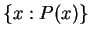) Let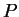be a proposition form over a set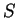, and let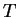be a subset of. Then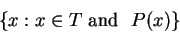(3.25)

is defined to be the set of all elements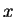insuch that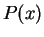is true. The set described in (3.25) is also written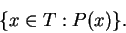In cases where the meaning of'' is clear from the context, we may abbreviate (3.25) by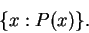3.26   Examples.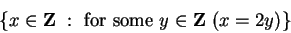is the set of all even integers, and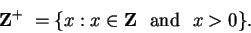If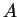and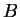are sets, then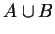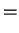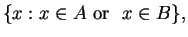(3.27)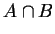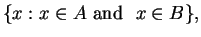(3.28)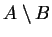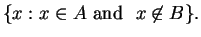(3.29)

We will use the following notation throughout these notes.

3.30   Notation (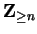,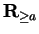) If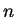is an integer we define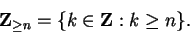ThusSimilarly, if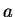is a real number, we define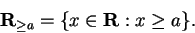3.31   Definition (Ordered pair.) If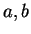are objects, then the ordered pair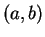is a new object obtained by combining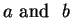. Two ordered pairs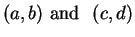are equal if and only if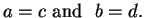Similarly we may consider ordered triples. Two ordered triples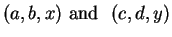are equal if and only if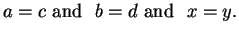We use the same notationto represent an open interval in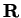and an ordered pair in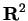. The context should always make it clear which meaning is intended.

3.32   Definition (Cartesian product) If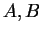are sets then the Cartesian product ofandis defined to be the set of all ordered pairs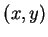such that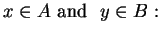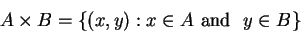(3.33)

3.34   Examples. Let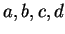be real numbers with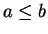and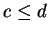. Then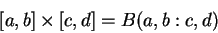and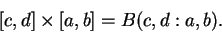Thus in general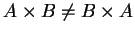.

The set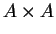is denoted by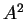. You are familiar with one Cartesian product. The euclidean planeis the Cartesian product of R with itself.

3.35   Exercise. Let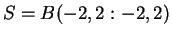and let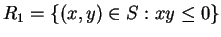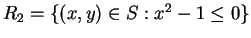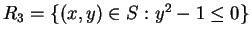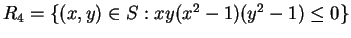Sketch the sets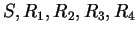. For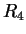you should include an explanation of how you arrived at your answer. For the other sets no explanation is required.

3.36   Exercise. Do there exist setssuch that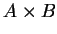has exactly five elements?Next: 3.3 Functions Up: 3. Propositions and Functions Previous: 3.1 Propositions   Index
Ray Mayer 2007-09-07